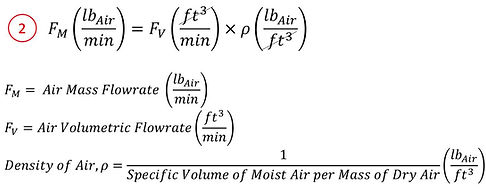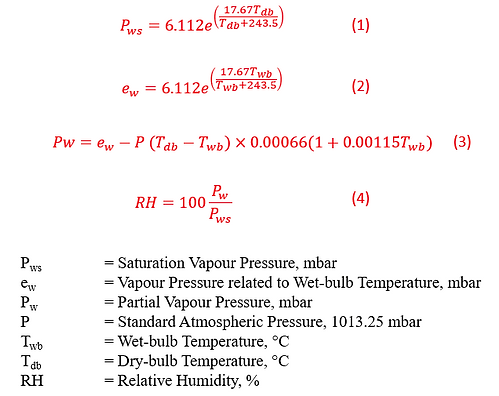AC Condensate Flowrate Calculator

The Condensate Water Flowrate of an Air-Conditioning Unit can be determined by how much moisture the Cooling Coil can remove from the Air at a given time.

The following steps can be used to calculate the condensate flowrate.

1. Calculating the difference in the Humidity Ratio between the On-Coil and the Off-Coil Air. Humidity Ratio is the ratio of the weight of water vapour (lb) contained in an air sample to the weight of the dry air (lb). This will tell us how much moisture content the cooling coil has removed from the Entering Air.2. Convert Volumetric Air Flowrate, FV (cfm) to Mass Air Flowrate, FM (lb/min), by multiplying with density of air, ρ – from a given Volumetric Air Flowrate (ft3/min), determine the weight of air that pass through the cooling coil at a given time (lb/min). Density of air, ρ at the cooling coil can also be derived from the specific volume of moist air per mass of dry air, which can in turn be derived from the psychrometric chart (or any online psychrometric calculators). It depends on Temperature and Pressure.3. With the difference in Humidity Ratio (lbH2O/lbDry Air) and the mass flow rate of air (lb/ft3), we can derive the condensate flowrate, CM from the formula below.4. To Convert lb/min to usgpm, multiply the result by 0.12.Example:

Calculate the Condensate Flowrate in usgpm for the following (FV=5,000cfm):The Humidity Ratio of the Entering Air Temperature and Leaving Air Temperature can be determined from the psychrometric chart (or an online psychrometric calculator) with the given dry bulb and wet bulb temperature.It is noticeable that information that include the humidity ratio, the specific volume of dry air etc. can be derived from a psychrometric chart. On the other hand, an online psychrometric calculator can also calculate the properties using formulae. A downloadable Excel is also available below containing the following formulae.

Condensate Flowrate Calculator (Excel)

The Humidity Ratio of the Entering Air Temperature and Leaving Air Temperature can be determined from the psychrometric chart (or an online psychrometric calculator) with the given dry bulb and wet bulb temperature.

Some of these formulae for the computation of Water Vapour Saturation Pressure (Pws), Water Vapour Partial Pressure (Pw),the Humidity Ratio (HR), density or specific volume, have been further elaborated in the page: Advanced Psychrometric Calculator. For ease of reference, they are being extracted as follows: